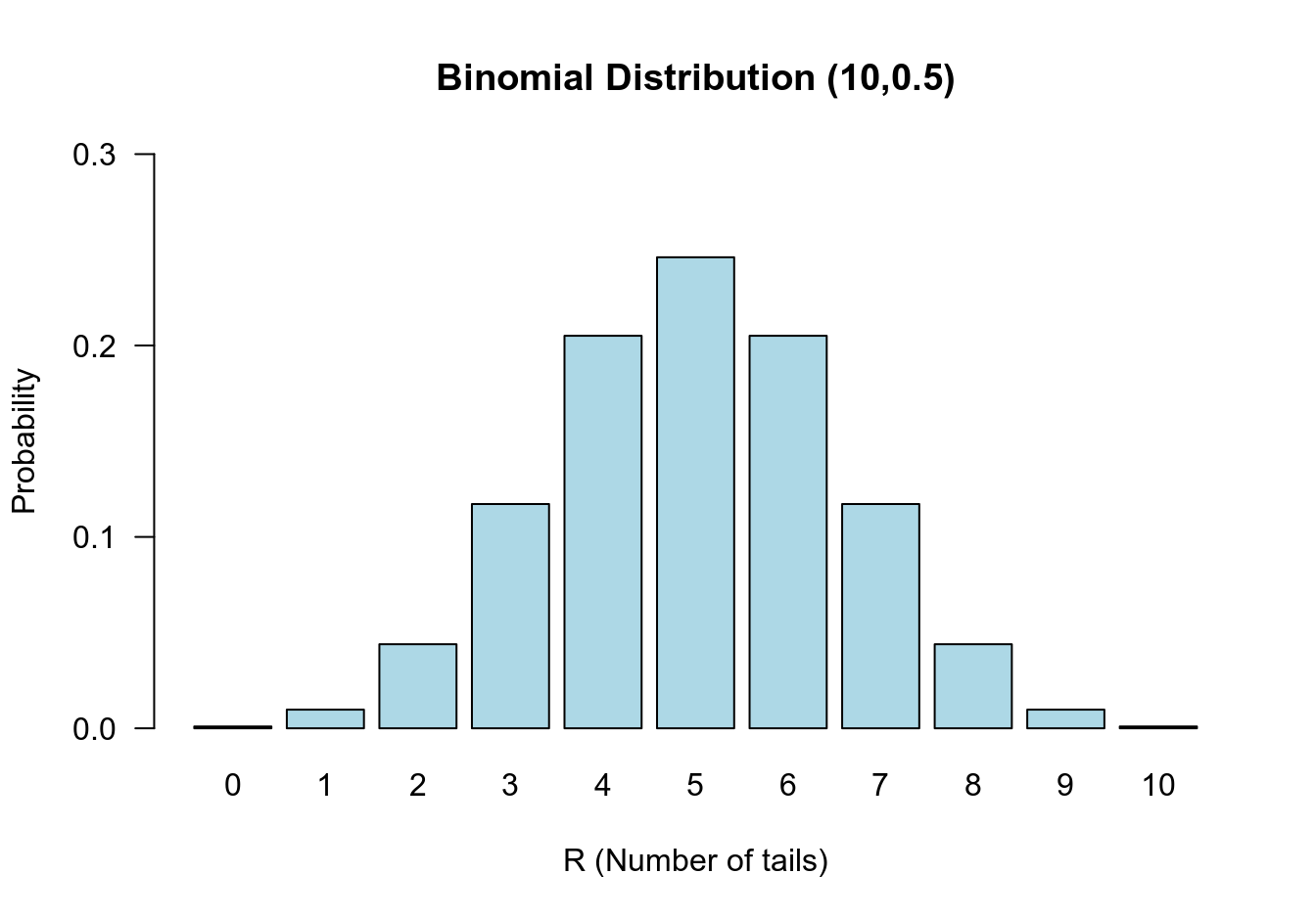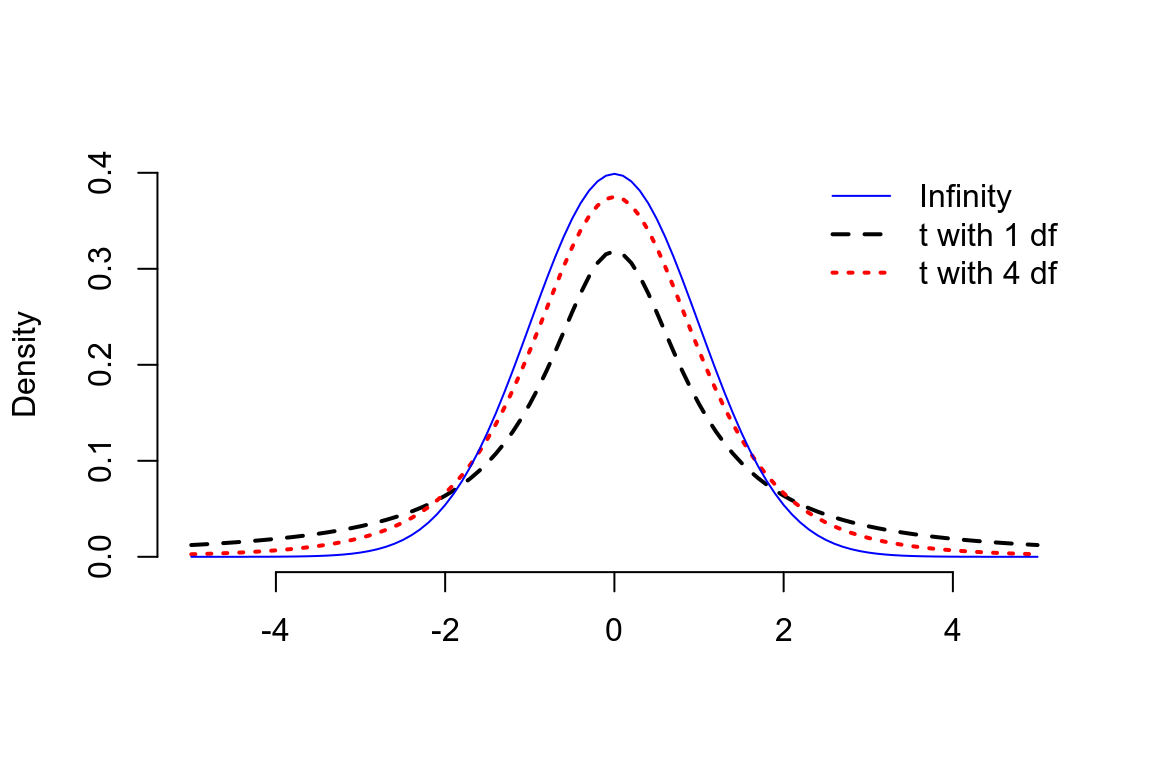# 第 22 章 假設檢驗

## 22.1 拋硬幣的例子

• “一次反面朝上”，
• “零次反面朝上”，
• “九次反面朝上 (或者說一次正面朝上)”，
• “十次反面朝上 (或者說零次正面朝上)”。

• “兩次反面朝上”，
• “三次反面朝上”，
• “四次反面朝上”，
• “五次反面朝上”，
• “六次反面朝上”，
• “七次反面朝上”，
• “八次反面朝上”。

$\begin{equation} \text{Prob}\{ R \text{ as or more extreme than } r | \pi=0.5 \} \end{equation} \tag{22.1}$\begin{aligned} &\text{Prob} \{R=0|\pi=0.5\} + \text{Prob} \{R=1|\pi=0.5\} + \text{Prob} \{R=9|\pi=0.5\} + \text{Prob} \{R=10|\pi=0.5\} \\ & = (\binom{10}{0} + \binom{10}{1} + \binom{10}{9} + \binom{10}{10})\times(0.5)^{10} = 0.021 \end{aligned}

### 22.1.1 單側和雙側檢驗

\begin{aligned} &\text{Prob}\{R\leqslant r| \pi=0.5\}\\ &\text{In the example } r=1 \\ &\Rightarrow \text{Prob}\{R=0 | \pi = 0.5\} + \text{Prob}\{ R=1 | \pi = 0.5 \} = 0.011 \end{aligned}

## 22.2 二項分佈的精確假設檢驗

$$n$$ 次實驗中成功次數爲 $$R$$，那麼樣本百分比 (估計，estimator) $$P=\frac{R}{n}$$ 是它的人羣比例 $$\pi$$ (參數，parameter) 的無偏估計。欲檢驗的零假設 $$\pi=\pi_0$$，替代假設 $$\pi\neq\pi_0$$，且某一次觀察結果爲 $$R=r$$，我們要計算的 $$p$$ 值就是在零假設條件下，所有情況中 $$R=r$$ 或者與之同等極端甚至更加極端的事件所佔的比例。

• 如果 $$r<n\pi_0$$，單側 $$p$$ 值等於

\begin{aligned} p & = \text{Prob}\{ r\text{ or fewer successes out of n | \pi=\pi_0} \} \\ & = P_0 + P_1 + P_2 + \cdots + P_r \\ \text{Where } & P_x = \binom{n}{x} \pi_0^x (1-\pi_0)^{n-x} \end{aligned}

• 如果 $$r>n\pi_0$$，單側 $$p$$ 值等於

\begin{aligned} p & = \text{Prob}\{ r\text{ or more successes out of n | \pi=\pi_0} \} \\ & = P_r + P_{r+1} + P_{r+2} + \cdots + P_{n} \\ \text{Where } & P_x = \binom{n}{x} \pi_0^x (1-\pi_0)^{n-x} \end{aligned}

## 22.3 當樣本量較大

$\begin{equation} Z=\frac{R-E(R)}{\sqrt{\text{Var}(R)}} = \frac{R-E(R)}{\text{SE}(R)} \end{equation} \tag{22.2}$

## 22.4 二項分佈的正態近似法假設檢驗

$E(R) = n\pi_0; \text{ and Var}(R) = n\pi_0(1-\pi_0)$

\begin{aligned} Z & = \frac{R-E(R)}{\sqrt{\text{Var}(R)}} \\ & = \frac{R-n\pi_0}{\sqrt{n\pi_0(1-\pi_0)}} \\ & = \frac{P-\pi_0}{\sqrt{\frac{\pi_0(1-\pi_0)}{n}}} \\ \text{Where } & P=\frac{R}{n} \end{aligned}

### 22.4.1 連續性校正 continuity correction

$Z=\frac{|R-n\pi_0|-\frac{1}{2}}{\sqrt{n\pi_0(1-\pi_0)}} \text{ or } Z=\frac{|P-\pi_0|-\frac{1}{2n}}{\sqrt{\frac{\pi_0(1-\pi_0)}{n}}}$

“Statistical Methods in Medical Research” (Armitage, Berry, and Matthews 2008) 書中建議，滿足 $$n\pi \geqslant 10 \text{ or } n(1-\pi) \geqslant 10$$ 時近似法計算的 $$p$$ 值可以給出較爲滿意的結果。另外，當 $$n>100$$ 則建議不再進行連續性校正，即把校正部分的 $$-\frac{1}{2}$$ 或者 $$-\frac{1}{2n}$$ 去掉。

## 22.5 情況1：對均值進行假設檢驗 (方差已知)

$\text{H}_0: \mu=\mu_0 \text{ v.s. H}_1: \mu\neq\mu_0$

$Z = \frac{\bar{Y}-E(\bar{Y})}{\sqrt{\text{Var}\bar{Y}}} = \frac{\bar{Y}-\mu_0}{\sqrt{\sigma^2/n}}$

\begin{aligned} p &= \text{Prob}(\bar{Y}\leqslant\bar{y}|\mu=\mu_0) \\ &= \text{Prob}(Z<\frac{\bar{y}-\mu_0}{\sqrt{\sigma^2/n}}) \\ &= \Phi(\frac{\bar{y}-\mu_0}{\sqrt{\sigma^2/n}}) \\ \text{Where } & \Phi \text{ is the distribution function for a } N(0,1) \text{distribution} \end{aligned}

$\text{Two-sided } p \text{ value } = 2\times[1-\Phi(\frac{\bar{y}-\mu_0}{\sqrt{\sigma^2/n}})]$

## 22.6 情況2：對均值進行假設檢驗 (方差未知) the one-sample t-test

$\text{H}_0: \mu=\mu_0 \text{ v.s. H}_1: \mu\neq\mu_0$

$T = \frac{\bar{Y}-\mu_0}{\sqrt{\hat\sigma^2/n}} \sim t_{n-1}$## 22.7 情況3：對配對實驗數據的均值差進行假設檢驗 the paired t-test

### References

Armitage, Peter, Geoffrey Berry, and John Nigel Scott Matthews. 2008. Statistical Methods in Medical Research. John Wiley & Sons.